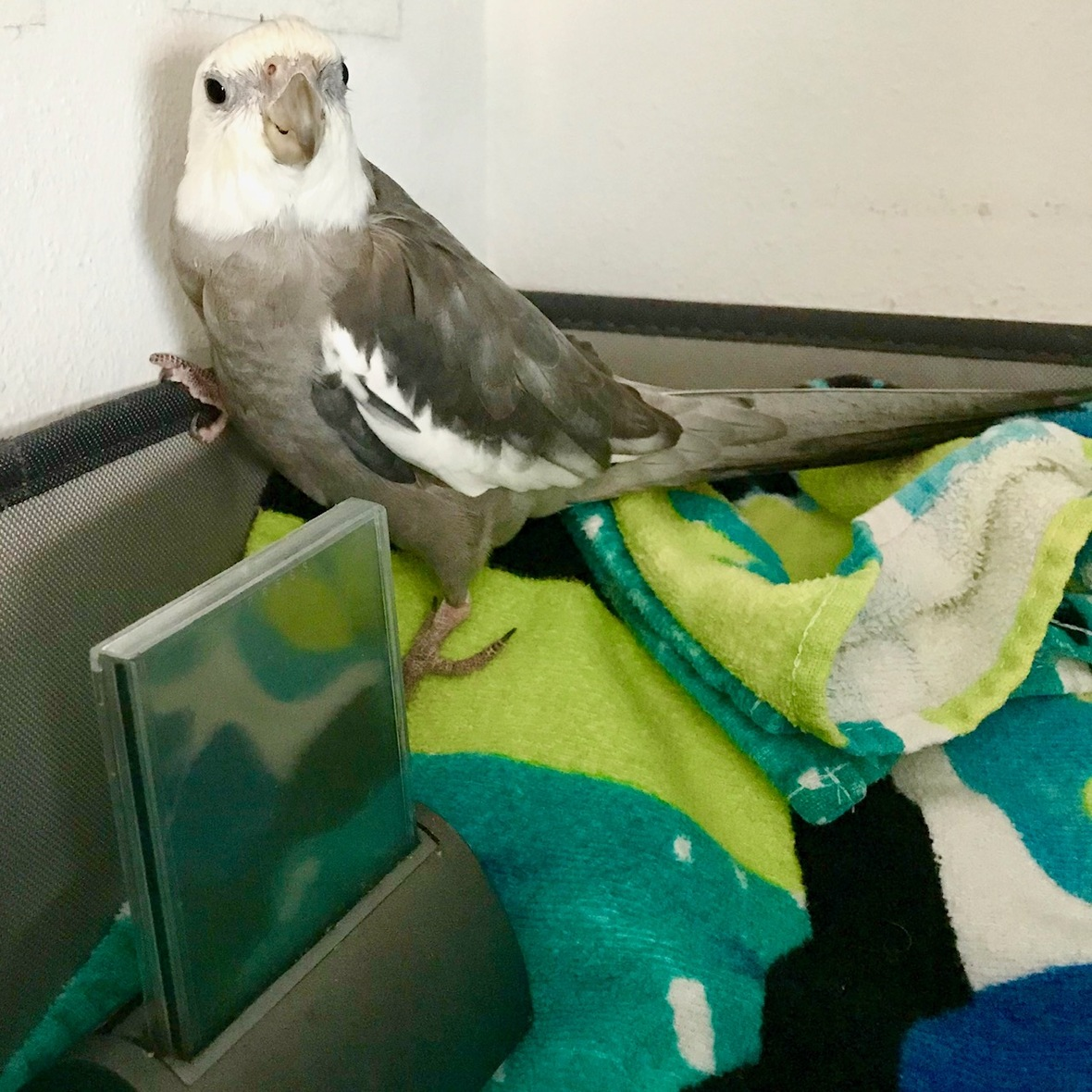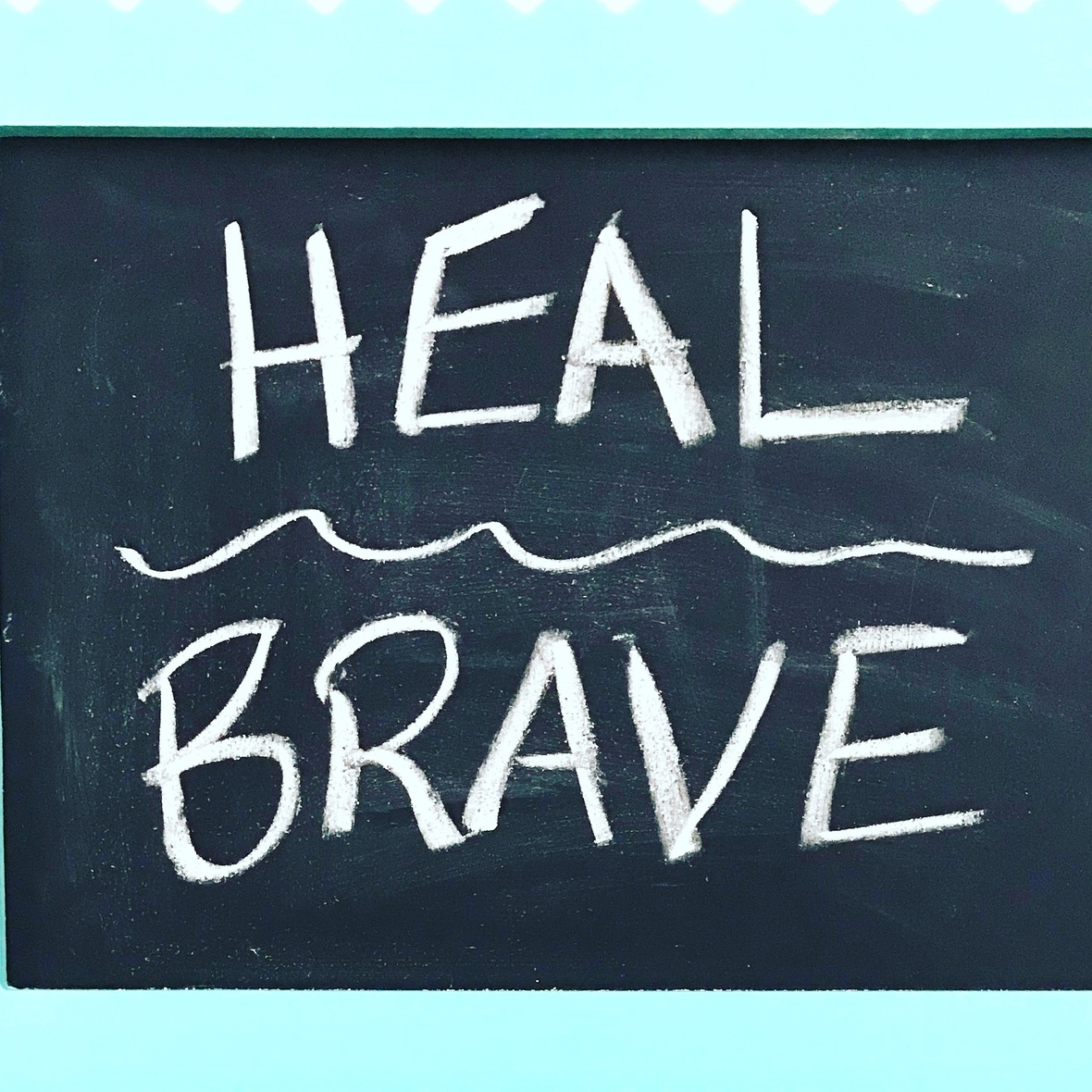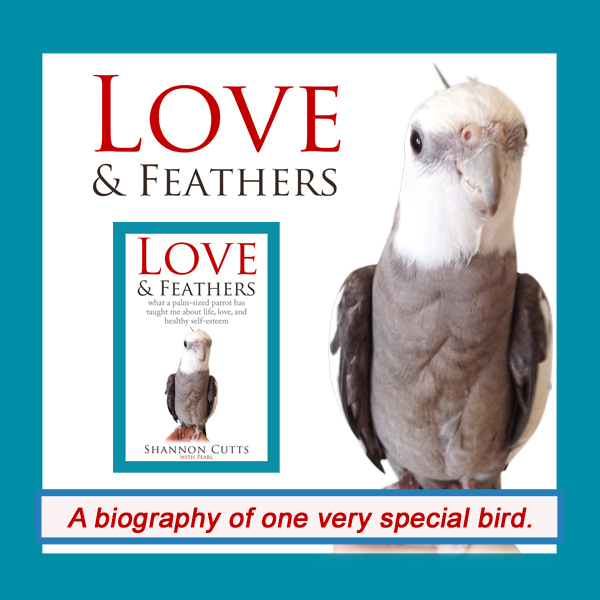# March 2019

 table div table+table+table div table{width:100%;padding:0}table div table+table+table div table img{width:96.23%;padding:0;float:none}table div table+table+table div table td{width:100%;padding:0 1.88% 18px}/* styles *//* styles */ Receiving this email as a forward? Sign up to keep following our little flock's adventures!
 table div table+table+table+table+table+table div table{width:100%;padding:0}table div table+table+table+table+table+table div table img{width:96.23%;padding:0;float:none}table div table+table+table+table+table+table div table td{width:100%;padding:0 1.88% 18px}/* styles */## Building Up Your Tolerance for Self-CareUnlike his mommy, my parrot, Pearl, has zero problem stopping everything for (even more) self-care. Here, he rests in his towel basket with his bestie, the bathroom clock, while his mommy does yoga nearby.

If you are like me, you probably read the title to this post and thought:

"Huh? What do you mean, tolerance for self-care? Shouldn't it be easy, enjoyable, fun even?"

Absolutely.

It sure should be easy, enjoyable, embraceable, fun. Yet, for so many (or at least for me) it isn't. Or it hasn't been. Until now.

This month, it is a little over a year since I was diagnosed with hypothyroidism (translation: thyroid decides to go on indefinite vacation, tells no one before it leaves.)

When I first was diagnosed, I was at a total loss. I mean, I knew something in me was broken. I felt it in my body, my mind, my emotions, my spirit. When my doctor told me at that first appointment not to worry, he could fix it, no matter what "it" was, I felt relieved. That was just what I wanted and needed to hear at the time.

But the thyroid pills only got me so far. And there were side effects. I knew more would be needed than just the medicine if I was to truly heal, but I didn't know how much more. And I didn't know what.

Today, I do. Today, I catch myself saying to myself, "I just need a 'me' day, a 'spa' day, a 'self-care' day," so much more often and usually without more than a twinge of guilt. Sometimes I even say it out loud to myself, to Pearl, to Malti, to Bruce, to the air around me that seems empty but isn't.

It is full of my energy, my effort, my emerging intuition that all goodness, all kindness, all compassion, all empathy, all joy, all love, all fun, all connection, all that is really alive begins within.

Today I am not as productive in the ways I used to define productivity. I have creative projects that are - dare I say it - languishing - so I can take yet another hour to give myself a pedicure or a clay masque or a yoga session.

They might never get done, to be honest.

I mean, I hope they will get done and I want them to get done and I haven't given up on getting them done. But right now and likely for the foreseeable future, the one that is going to do them is the same one that needs more TLC and self-care than she ever has before, and by golly she is going to get it.

And I have to say, my thyroid gland is flat-out basking in the glow of all this attention.

My other body parts, which at first were fairly equal parts resentful and jealous of it, have now jumped on board. After all, when one organ gets "discovered" and all the fruits of that newfound fame come pouring in, every organ, every part, every body system also cashes in on the bounty.

More nourishment. More rest. More yoga. More good books. More time with the flock. More meditation. More patience. More trust. More healing and health and restoration for all.

I feel it is also so important to point out that, this time, when I was first diagnosed with hypothyroidism, I wasn't starting from scratch with learning to do the hard and rewarding work of recovery itself.

After all, I already had two decades of valuable (dare I say life-saving) recovery experience and skills I had gained from battling anorexia, bulimia, anxiety and depression. These skills and experiences are getting used again daily now! They matter!

This is why, about mid-way through my earlier recovery journey, I suddenly realized that the specific diagnosis doesn't really matter. I mean, it does, because it can inform what you do and who you connect with and how you proceed. But it also doesn't, because everyone has something.

Everyone struggles with something. At that most fundamental level, we truly are all in this together.

Today, in mid-process with yet another serious health issue that needs my full recovery attention, I know for a fact that this is true.

As I began battling my way back from thyroid dysfunction, I hung a small chalkboard on my wall near my yoga mat.

I wrote these words: "Heal. Brave."

Those words are still there, over a year later. I look at them several times a day. They remind me what we are doing here, me and myself and I, on the mat, off the mat, inside me, around me, in my own life.

That little chalkboard and its two words help me every day to invest and commit and reinvest and recommit to self-care, to self-kindness, to self-compassion, to self-uncovering, to wake up for one more day just to see what might happen next.

Over the last year, I have transformed from the inside out. I feel older, wiser, yet younger, newer. I have learned new skills, discovered new truths, revitalized old beloved practices, unfolded a deeper layer of self, and in that process discovered someone with real substance who really wants to be here and live and try.

Building my tolerance for self-care has not been easy. It has not come willingly. I do not feel I was born into a culture of, "Self-love! Yay!" "Self-care! Good!" Just the opposite, really.

So I have had to seek out self-care mentors, guides, friends, teachers, wise lights a few steps or many miles ahead of me on this initially dimly lit path that grows brighter the longer I walk along it.

At my core, I have had to choose whether to develop myself in this area. Truthfully, it would have been easier in many ways to choose not to.

For instance, I could have kept my old toxic friendships (including my old toxic pseudo-friendship with myself).

I could have stayed the course in my harmful eating and drinking habits that often felt good in the moment but always hurt me a lot later on.

I could have believed my own excuses when I said I "had to" get such-and-so to-do list task done and that meant I didn't have 20 minutes to do yoga or an extra hour in the mornings to meditate.

I could have given up on myself at any point and called it "necessity" or "bad luck" or "the way things have to be."

Upon hearing this new diagnosis, I could have gone over to the dark side once again, to the same place where I spent all those many years trying to change my outsides so I could win acceptance and love and success from the cold, hard world around me - a world that was far too busy with its own pain to even notice or care about mine.

But (long story short), I didn't. I couldn't. Thank goodness.

Thanks to my previous and still-ongoing recovery journey through eating disorders and depression/anxiety, I knew everything I needed to know about where that road leads me. I already knew retreating back in that direction again wouldn't work.

I also knew this was my moment - my big chance - to learn to love myself, lumps, bumps, bruises, character flaws, oh-so-not-shining-moments and all.

This was my chance. This is my chance. I'm taking it. I would love to have you join me!

with great love and respect,

Shannon

 table div table+table+table+table+table+table+table+table+table div table{width:100%;padding:0}table div table+table+table+table+table+table+table+table+table div table img{width:96.23%;padding:0;float:none}table div table+table+table+table+table+table+table+table+table div table td{width:100%;padding:0 1.88% 18px}/* styles *//* styles */ If someone walked up to you and asked for advice about self-care, what would you tell them? What does self-care look like in your life? How do you know when you are taking good care of yourself...and when you aren't? I'd love to hear your insights!Yup. That's it. My Heal/Brave chalkboard hanging on the wall near where I do yoga each day.
 table div table+table+table+table+table+table+table+table+table+table+table+table div table{width:100%;padding:0}table div table+table+table+table+table+table+table+table+table+table+table+table div table img{width:96.23%;padding:0;float:none}table div table+table+table+table+table+table+table+table+table+table+table+table div table td{width:100%;padding:0 1.88% 18px}/* styles */# "Waffles & More" - Order Your Tasty Copy Today!

 table.module-13{width:75.47%;padding:0}table div table+table+table+table+table+table+table+table+table+table+table+table+table+table div table{width:75.47%;float:none;margin-left:auto;margin-right:auto;padding:0}table div table+table+table+table+table+table+table+table+table+table+table+table+table+table div table a{border:0 none;text-decoration:none}table div table+table+table+table+table+table+table+table+table+table+table+table+table+table div table img{width:100%!important;border:0 none;text-decoration:none}table div table+table+table+table+table+table+table+table+table+table+table+table+table+table div table td{width:100%;padding:0}/* styles */
 table div table+table+table+table+table+table+table+table+table+table+table+table+table+table+table+table div table{width:100%;padding:0}table div table+table+table+table+table+table+table+table+table+table+table+table+table+table+table+table div table img{width:96.23%;padding:0;float:none}table div table+table+table+table+table+table+table+table+table+table+table+table+table+table+table+table div table td{width:100%;padding:0 1.88% 18px}/* styles */# Get your copy of Love & Feathers the book!Click on the image of Pearl to order your signed copy!Pearl will even beak-o-graph it for you!
 table div table+table+table+table+table+table+table+table+table+table+table+table+table+table+table+table+table+table+table+table+table div table{width:100%;padding:0}table div table+table+table+table+table+table+table+table+table+table+table+table+table+table+table+table+table+table+table+table+table div table img{width:96.23%;padding:0;float:none}table div table+table+table+table+table+table+table+table+table+table+table+table+table+table+table+table+table+table+table+table+table div table td{width:100%;padding:0 1.88% 18px}/* styles */# Malti's fav self-care spot: with her alligator!

 table.module-22{width:75.47%;padding:0}table div table+table+table+table+table+table+table+table+table+table+table+table+table+table+table+table+table+table+table+table+table+table+table div table{width:75.47%;float:none;margin-left:auto;margin-right:auto;padding:0}table div table+table+table+table+table+table+table+table+table+table+table+table+table+table+table+table+table+table+table+table+table+table+table div table a{border:0 none;text-decoration:none}table div table+table+table+table+table+table+table+table+table+table+table+table+table+table+table+table+table+table+table+table+table+table+table div table img{width:100%!important;border:0 none;text-decoration:none}table div table+table+table+table+table+table+table+table+table+table+table+table+table+table+table+table+table+table+table+table+table+table+table div table td{width:100%;padding:0}/* styles */
 table div table+table+table+table+table+table+table+table+table+table+table+table+table+table+table+table+table+table+table+table+table+table+table+table+table div table{width:100%;padding:0}table div table+table+table+table+table+table+table+table+table+table+table+table+table+table+table+table+table+table+table+table+table+table+table+table+table div table img{width:96.23%;padding:0;float:none}table div table+table+table+table+table+table+table+table+table+table+table+table+table+table+table+table+table+table+table+table+table+table+table+table+table div table td{width:100%;padding:0 1.88% 18px}/* styles */# So eager for my sleepyhead boy to wake up!

 table.module-26{width:75.47%;padding:0}table div table+table+table+table+table+table+table+table+table+table+table+table+table+table+table+table+table+table+table+table+table+table+table+table+table+table+table div table{width:75.47%;float:none;margin-left:auto;margin-right:auto;padding:0}table div table+table+table+table+table+table+table+table+table+table+table+table+table+table+table+table+table+table+table+table+table+table+table+table+table+table+table div table a{border:0 none;text-decoration:none}table div table+table+table+table+table+table+table+table+table+table+table+table+table+table+table+table+table+table+table+table+table+table+table+table+table+table+table div table img{width:100%!important;border:0 none;text-decoration:none}table div table+table+table+table+table+table+table+table+table+table+table+table+table+table+table+table+table+table+table+table+table+table+table+table+table+table+table div table td{width:100%;padding:0}/* styles */
 table div table+table+table+table+table+table+table+table+table+table+table+table+table+table+table+table+table+table+table+table+table+table+table+table+table+table+table+table+table div table{width:100%;padding:0}table div table+table+table+table+table+table+table+table+table+table+table+table+table+table+table+table+table+table+table+table+table+table+table+table+table+table+table+table+table div table img{width:96.23%;padding:0;float:none}table div table+table+table+table+table+table+table+table+table+table+table+table+table+table+table+table+table+table+table+table+table+table+table+table+table+table+table+table+table div table td{width:100%;padding:0 1.88% 18px}/* styles */## Meet Pearl, Malti, Bruce & Me

 table div table+table+table+table+table+table+table+table+table+table+table+table+table+table+table+table+table+table+table+table+table+table+table+table+table+table+table+table+table+table+table div table,table.module-30{width:34.34%;float:left;padding:0}table div table+table+table+table+table+table+table+table+table+table+table+table+table+table+table+table+table+table+table+table+table+table+table+table+table+table+table+table+table+table+table div table a{border:0 none;text-decoration:none}table div table+table+table+table+table+table+table+table+table+table+table+table+table+table+table+table+table+table+table+table+table+table+table+table+table+table+table+table+table+table+table div table img{width:100%!important;border:0 none;text-decoration:none}table div table+table+table+table+table+table+table+table+table+table+table+table+table+table+table+table+table+table+table+table+table+table+table+table+table+table+table+table+table+table+table div table td{width:100%;padding:0 20px 20px 0}/* styles */ Hi! It is so good to meet you here each month! I'm so excited about our newest book, "Waffles & More: A Love & Feathers Recipe Book," which features so many of the recipes our flock (and especially the flock member with the feathers) particularly enjoys! And of course there is the book that started it all - "Love & Feathers: what a palm-sized parrot has taught me about life, love, and healthy self-esteem." This fun and feathery book focuses on the power of animal mentors in our lives and features my life with my precious parrot, Pearl. Malti is my darling young red-foot tortoise. She has such a healthy appetite for food, snoozing and life! Bruce, a very brave rescued Texas 3-toed box turtle, joined our flock a few years ago in a space clearly reserved just for him! My first book, "Beating Ana: how to outsmart your eating disorder and take your life back," is a practical hands-on workbook to help overcome eating disorders as well as the story of my personal recovery journey. MentorCONNECT, the first global eating disorders nonprofit mentoring community, emerged from the experience of writing "Beating Ana." While we closed in April 2017, the website continues to provide information about mentoring-based recovery support resources. ==> To connect with me: www.shannoncutts.com ==> To connect with Pearl, Malti & Bruce: www.loveandfeathersandshells.com
 table div table+table+table+table+table+table+table+table+table+table+table+table+table+table+table+table+table+table+table+table+table+table+table+table+table+table+table+table+table+table+table+table div table{width:100%;padding:0}table div table+table+table+table+table+table+table+table+table+table+table+table+table+table+table+table+table+table+table+table+table+table+table+table+table+table+table+table+table+table+table+table div table img{width:96.23%;padding:0;float:none}table div table+table+table+table+table+table+table+table+table+table+table+table+table+table+table+table+table+table+table+table+table+table+table+table+table+table+table+table+table+table+table+table div table td{width:100%;padding:0 1.88% 18px}/* styles */table div table+table+table+table+table+table+table+table+table+table+table+table+table+table+table+table+table+table+table+table+table+table+table+table+table+table+table+table+table+table+table+table+table div table td,table.module-32{width:100%;padding:0}table div table+table+table+table+table+table+table+table+table+table+table+table+table+table+table+table+table+table+table+table+table+table+table+table+table+table+table+table+table+table+table+table+table div table{width:100%;float:none;margin-left:auto;margin-right:auto;padding:0}table div table+table+table+table+table+table+table+table+table+table+table+table+table+table+table+table+table+table+table+table+table+table+table+table+table+table+table+table+table+table+table+table+table div table a{border:0 none;text-decoration:none}table div table+table+table+table+table+table+table+table+table+table+table+table+table+table+table+table+table+table+table+table+table+table+table+table+table+table+table+table+table+table+table+table+table div table img{width:100%!important;border:0 none;text-decoration:none}/* styles */
 table.module-33{width:74.15%;padding:0}table div table+table+table+table+table+table+table+table+table+table+table+table+table+table+table+table+table+table+table+table+table+table+table+table+table+table+table+table+table+table+table+table+table+table div table{width:74.15%;float:none;margin-left:auto;margin-right:auto;padding:0}table div table+table+table+table+table+table+table+table+table+table+table+table+table+table+table+table+table+table+table+table+table+table+table+table+table+table+table+table+table+table+table+table+table+table div table a{border:0 none;text-decoration:none}table div table+table+table+table+table+table+table+table+table+table+table+table+table+table+table+table+table+table+table+table+table+table+table+table+table+table+table+table+table+table+table+table+table+table div table img{width:100%!important;border:0 none;text-decoration:none}table div table+table+table+table+table+table+table+table+table+table+table+table+table+table+table+table+table+table+table+table+table+table+table+table+table+table+table+table+table+table+table+table+table+table div table td{width:100%;padding:0}/* styles */
 table div table+table+table+table+table+table+table+table+table+table+table+table+table+table+table+table+table+table+table+table+table+table+table+table+table+table+table+table+table+table+table+table+table+table+table div table{width:100%;padding:0}table div table+table+table+table+table+table+table+table+table+table+table+table+table+table+table+table+table+table+table+table+table+table+table+table+table+table+table+table+table+table+table+table+table+table+table div table img{width:96.23%;padding:0;float:none}table div table+table+table+table+table+table+table+table+table+table+table+table+table+table+table+table+table+table+table+table+table+table+table+table+table+table+table+table+table+table+table+table+table+table+table div table td{width:100%;padding:0 1.88% 18px}/* styles */## Reprints & Reposts

REPRINTS: Would you like to use any portion of this material in your newsletter, blog or website? You may do so as long as you also include this copyright information: